ML Aggarwal Class 10 Solutions for ICSE Maths Chapter 20 Heights and Distances Chapter Test

ML Aggarwal Class 10 Solutions for ICSE Maths Chapter 20 Heights and Distances Chapter Test

ML Aggarwal Class 10 Solutions for ICSE Maths Chapter 20 Heights and Distances Chapter Test

Question 1.
The angle of elevation of the top of a tower from a point A (on the ground) is 30°. On walking 50 m towards the tower, the angle of elevation is found to be 60°. Calculate
(i) the height of the tower (correct to one decimal place).
(ii) the distance of the tower from A.
Solution: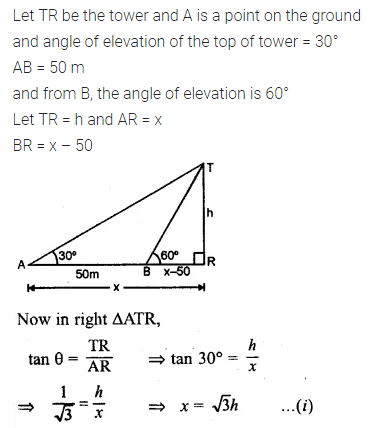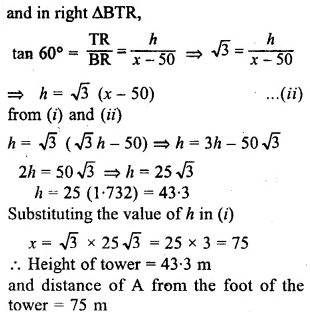Question 2.
An aeroplane 3000 m high passes vertically above another aeroplane at an instant when the angles of elevation of the two aeroplanes from the same point on the ground are 60° and 45° respectively. Find the vertical distance between the two planes.
Solution: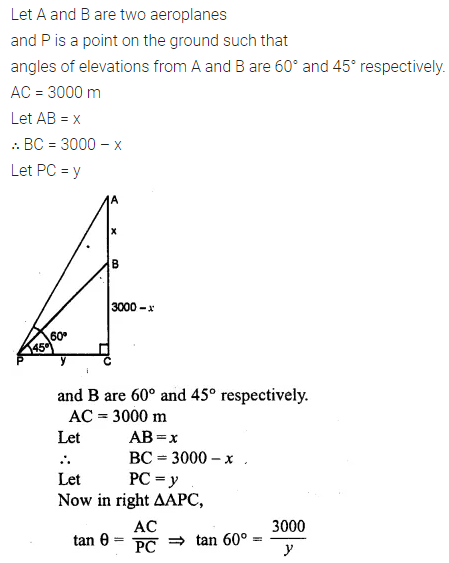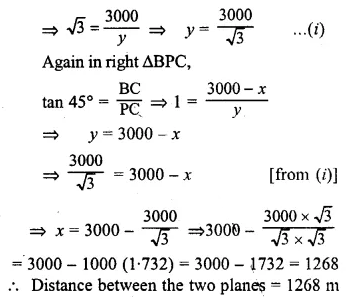Question 3.
A 7m long flagstaff is fixed on the top of a tower. From a point on the ground, the angles of elevation of the top and bottom of the flagstaff are 45° and 36° respectively. Find the height of the tower correct to one place of demical.
Solution: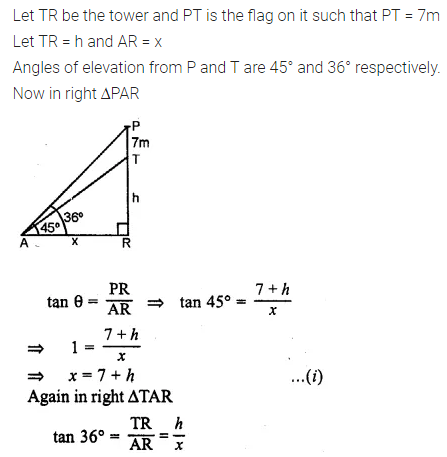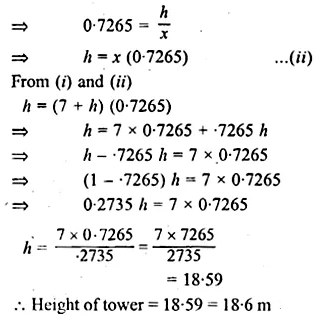Question 4.
A boy 1.6 m tall is 20 m away from a tower and observes that the angle of elevation of the top of the tower is 60°. Find the height of the tower.
Solution:Question 5.
A boy 1.54 m tall can just see the sun over a wall 3.64 m high which is 2.1 m away from him. Find the angle of elevation of the sun.
Solution: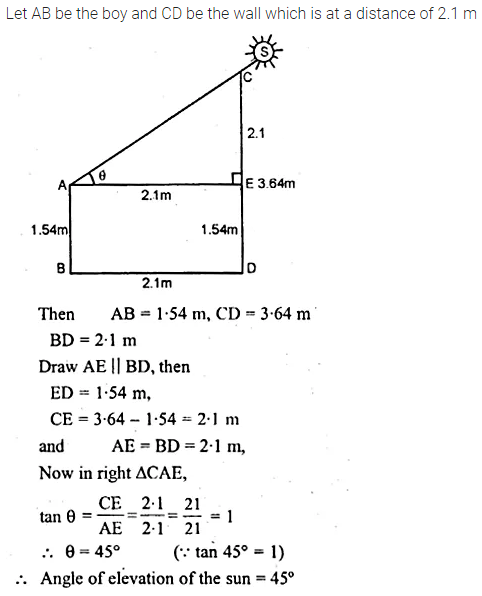Question 6.
In the adjoining figure, the angle of elevation of the top P of a vertical tower from a point X is 60° ; at a point Y, 40 m vertically above X, the angle of elevation is 45°. Find
(i) the height of the tower PQ
(ii) the distance XQSolution:Question 7.
An aeroplane is flying horizontally 1 km above the ground is observed at an elevation of 60°. After 10 seconds, its elevation is observed to be 30°. Find the speed of the aeroplane in km/hr.
Solution: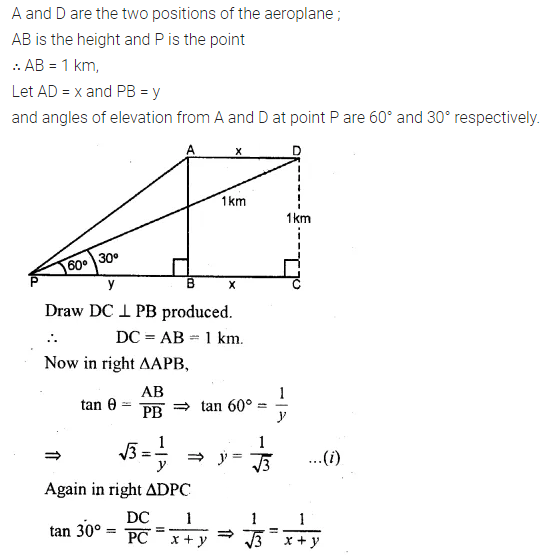Question 8.
A man on the deck of a ship is 16 m above the water level. He observes that the angle of elevation of the top of a cliff is 45° and the angle of depression of the base is 30°. Calculate the distance of the cliff from the ship and the height of the cliff.
Solution: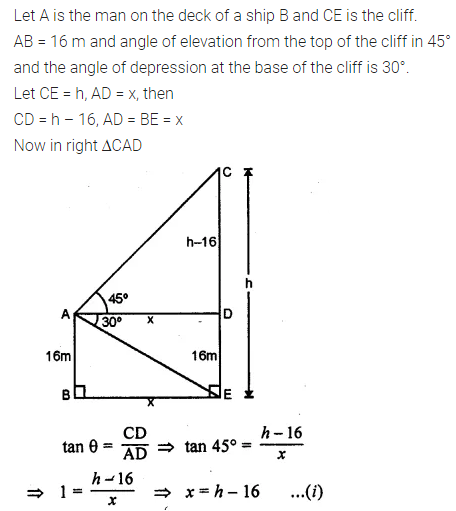Question 9.
There is a small island in between a river 100 metres wide. A tall tree stands on the island. P and Q are points directly opposite to each other on the two banks and in line with the tree. If the angles of elevation of the top of the tree from P and Q are 30° and 45° respectively, find the height of the tree.
Solution:Question 10.
A man standing on the deck of the ship which is 20 m above the sea-level, observes the angle of elevation of a bird as 30° and the angle of depression of its reflection in the sea as 60°. Find the height of the bird
Solution: